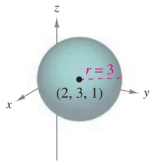Chapter 7.1, Problem 30E### Calculus: An Applied Approach (Min...

10th Edition
Ron Larson
ISBN: 9781305860919

#### Solutions

Chapter
Section### Calculus: An Applied Approach (Min...

10th Edition
Ron Larson
ISBN: 9781305860919
Textbook Problem
1 views

# Finding the equation of a Sphere In Exercises 29–38, find the standard equation of the sphere with the given characteristics. See Examples 4 and 5.To determine

To calculate: The standard equation of sphere from the provided figure,Explanation

Given Information:

The provided figure,

Formula used:

The standard equation of a sphere with centre at (h,k,l) and radius r is,

(xh)2+(yk)2+(zj)2=r2

Calculation:

From the provided figure, the centre of sphere is (2,3,1) and radius is 3.

The standard equation of a sphere,

(xh)2+(yk)2+(zj

### Still sussing out bartleby?

Check out a sample textbook solution.

See a sample solution

#### The Solution to Your Study Problems

Bartleby provides explanations to thousands of textbook problems written by our experts, many with advanced degrees!

Get Started

#### The graph at the right has equation:

Study Guide for Stewart's Multivariable Calculus, 8th

#### Solve for x: ln(e + x) = 1. a) 0 b) 1 c) e d) ee e

Study Guide for Stewart's Single Variable Calculus: Early Transcendentals, 8th

#### Identify the basic features of a between-subjects research design.

Research Methods for the Behavioral Sciences (MindTap Course List)